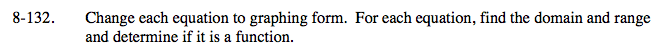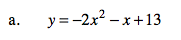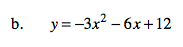### Home > A2C > Chapter 8 > Lesson 8.2.2 > Problem8-132

8-132.Factor the terms containing x so the coefficient of x² is 1, then complete the square.

$y=-2\left(x^{2}+\frac{1}{2}x\right)+13$

$y=-2\left(x^{2}+\frac{1}{2}x+\frac{1}{16}\right)-(-2)\left(\frac{1}{16}\right)+13$

$y=-2\left(x+\frac{1}{4}\right)^{2}+\frac{105}{8}$See part (a).